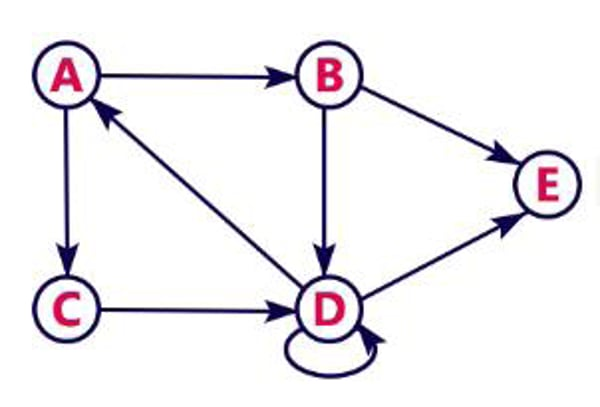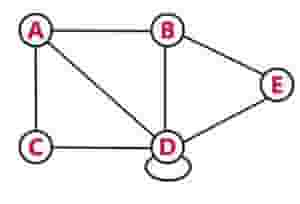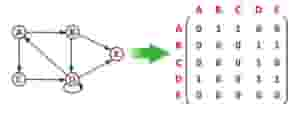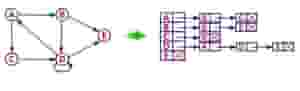Related Tags

data structures
python
graphs
community creator

# GRAPHS: basics, representation, traversals, and applications

Aruna kumari

## Basic concepts

### Definition

A graph G(V, E) is a non-linear data structure that consists of node and edge pairs of objects connected by links.

There are 2 types of graphs:

• Directed
• Undirected

### Directed graph

A graph with only directed edges is said to be a directed graph.

Example

The following directed graph has 5 vertices and 8 edges. This graph G can be defined as G = (V, E), where V = {A,B,C,D,E} and E = {(A,B), (A,C) (B, E), (B,D), (D, A), (D, E),(C,D),(D,D)}.Directed Graph

### Undirected graph

A graph with only undirected edges is said to be an undirected graph.

Example

The following is an undirected graph.Undirected Graph

## Representation of Graphs

Graph data structure is represented using the following representations.

• In this representation, the graph can be represented using a matrix of size n x n, where n is the number of vertices.
• This matrix is filled with either 1’s or 0’s.
• Here, 1 represents that there is an edge from row vertex to column vertex, and 0 represents that there is no edge from row vertex to column vertex.Directed graph representation

• In this representation, every vertex of the graph contains a list of its adjacent vertices.
• If the graph is not dense, i.e., the number of edges is less, then it is efficient to represent the graph through the adjacency list.## Graph traversals

• Graph traversal is a technique used to search for a vertex in a graph. It is also used to decide the order of vertices to be visited in the search process.
• A graph traversal finds the edges to be used in the search process without creating loops. This means that, with graph traversal, we can visit all the vertices of the graph without getting into a looping path. There are two graph traversal techniques:
1. DFS (Depth First Search)

### Procedure for graph traversal using DFS

    Step 1: Define a Stack of size total number of vertices in the
graph.

Step 2: Select any vertex as the starting point for traversal.
Visit that vertex and push it on to the Stack.

Step 3: Visit any one of the adjacent vertices of the vertex,
that is at top of the stack and is not visited, and
push it on to the stack.

Step 4: Repeat step 3 until there are no new vertices to visit
from each vertex on top of the stack.

Step 5: When there is no new vertex to visit, use
backtracking and pop one vertex from the stack.

Step 6: Repeat steps 3, 4, and 5 until stack becomes Empty.

Step 7: When stack becomes Empty, produce the final
spanning-tree by removing unused edges from the graph.


Python implementation

   #BFS method to traverse graph

def dfs_iterative(graph, start_vertex):
visited = set()
traversal = []
stack = [start_vertex]
while stack:
vertex = stack.pop()
if vertex not in visited:
traversal.append(vertex)
# add vertex in the same order as visited
stack.extend(reversed(graph[vertex]))
return traversal

test_graph = {
'A': ['B', 'S'],
'B': ['A'],
'C': ['D', 'E', 'F', 'S'],
'D': ['C'],
'E': ['C', 'H'],
'F': ['C', 'G'],
'G': ['F', 'S'],
'H': ['E', 'G'],
'S': ['A', 'C', 'G']
}

print(dfs_iterative(test_graph, 'A'))

### Procedure for graph traversal using BFS

    Step 1: Define a Queue of size total number of vertices in the
graph.

Step 2: Select any vertex as the starting point for traversal.
Visit that vertex and insert it into the Queue.

Step 3: Visit all the adjacent vertices of the vertex, that is
in front of the Queue and is not visited, and insert
them into the Queue.

Step 4: When there is no new vertex to visit from the vertex in
front of the Queue, delete that vertex from the
Queue.

Step 5: Repeat steps 3 and 4 until the queue becomes empty.

Step 6: When the queue becomes Empty, produce the final
spanning-tree by removing unused edges from the graph.

Python implementation

#BFS method to traverse graph

def bfs(graph, start):
path = []
queue = [start]
while queue:
vertex = queue.pop(0)
if vertex not in path:
path.append(vertex)
queue.extend(graph[vertex])
return path

test_graph = {
'A': ['B', 'S'],
'B': ['A'],
'C': ['D', 'E', 'F', 'S'],
'D': ['C'],
'E': ['C', 'H'],
'F': ['C', 'G'],
'G': ['F', 'S'],
'H': ['E', 'G'],
'S': ['A', 'C', 'G']
}
print(bfs(test_graph, 'A'))

## Applications of graphs

Since they are powerful abstractions, graphs can be very important in modeling data. In fact, many problems can be reduced to known graph problems. Here we outline just some of the many applications of graphs.

1. Social network graphs: To tweet or not to tweet. Graphs that represent who knows whom, who communicates with whom, who influences whom, or other relationships in social structures. An example is the twitter graph of who follows whom.

2. Graphs in epidemiology: Vertices represent individuals and directed edges to view the transfer of an infectious disease from one individual to another. Analyzing such graphs has become an important component in understanding and controlling the spread of diseases.

3. Protein-protein interactions graphs: Vertices represent proteins and edges represent interactions between them that carry out some biological function in the cell. These graphs can be used to, for example, study molecular pathway—chains of molecular interactions in a cellular process.

4. Network packet traffic graphs: Vertices are IP (Internet protocol) addresses and edges are the packets that flow between them. Such graphs are used for analyzing network security, studying the spread of worms, and tracking criminal or non-criminal activity.

5. Neural networks: Vertices represent neurons and edges are the synapses between them. Neural networks are used to understand how our brain works and how connections change when we learn. The human brain has about 1011 neurons and close to 1015 synapses.

RELATED TAGS

data structures
python
graphs
community creator

CONTRIBUTOR

Aruna kumari
RELATED COURSES

View all Courses

Keep Exploring

Learn in-demand tech skills in half the time